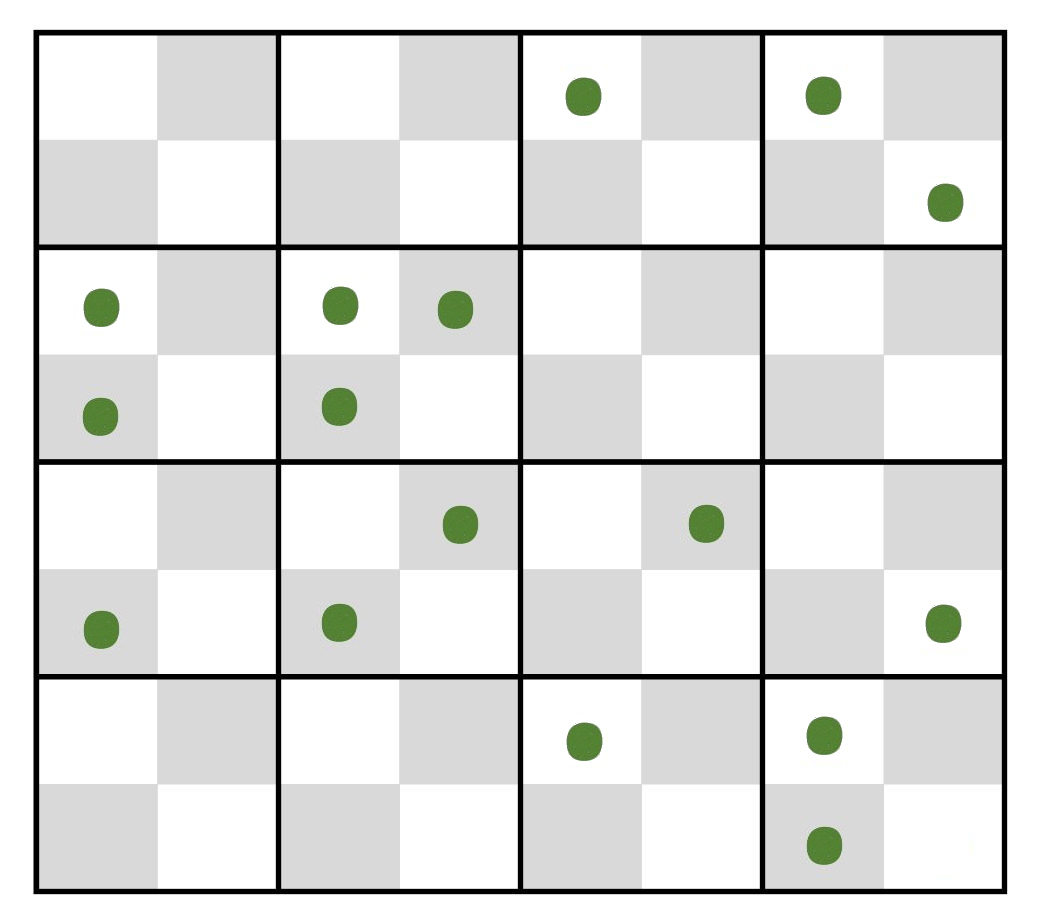µ  |  N 0 1 2 3 4 5 not 0 >(0+1) 0.100 90.5% 9.0% 0.5% 0.0% 0.0% 0.0% 9.5% 0.5% 0.125 88.2% 11.0% 0.7% 0.0% 0.0% 0.0% 11.8% 0.7% 0.250 77.9% 19.5% 2.4% 0.2% 0.0% 0.0% 22.1% 2.6% 0.500 60.7% 30.3% 7.6% 1.3% 0.2% 0.0% 39.3% 9.0% 0.750 47.2% 35.4% 13.3% 3.3% 0.6% 0.1% 52.8% 17.3% 1.000 36.8% 36.8% 18.4% 6.1% 1.5% 0.3% 63.2% 26.4%

The Poisson Distribution

The Poisson distribution is a special case of the binomial distribution that applies where the phenomenon under study occurs as rare, discrete events. The characteristic statistical property of a Poisson distribution is that the variance equals the mean (2 = µ). The probability P of observing Y events in a Poisson-distributed process with a mean = u is

P(Y; u) = e-u uY / Y!

(where Y! = Y factorial). This distribution provides a number of useful tools..

(1) In a study of the distribution of a rare plant among a number of standardized quadrat plots, a majority of plots may be expected to contain no specimens, a smaller number a single plant, and still smaller numbers two, three, or more plants. If 16 plants are distributed randomly over the 4x4 checkerboard quadrat below (mean µ = 1), the table shows that a random Poisson distribution over the cells should produce "0" and "1" classes at 37% each, a "2" class at 18%, the "3" class at 6%, and the more frequent classes will take up the remaining 2%. In the example, there are16 plants distributed as 6, 5, 4, and 1 cells with 0, 1, 2, and 3 plants, respectively. A Chi-square test that conforms to expected µ = 1 ± 1 indicate that the rare plant is distributed randomly.(2) The Poisson can simplify analysis of a simple "either / or" data set. In the quadrat example with µ = 1, the Poisson random expectation is that 37% of the quadrat plots will be unoccupied (0) and the remaining 63% occupied.(not 0). In a 2x2 test, a significant excess of empty cells means the plants are clumped, and a significant deficiency means the plant distribution is more uniform. The former might occur if suitable soil is patchily distributed, the latter if plants space themselves out to avoid competition for resources.

(3) Conversely, if it assumed that events occur randomly the number of observed events can be used to estimate the actual number of events. For example, suppose I am throwing rocks at a building with 100 windows. Initially, a good estimate of the number of thrown rocks is the count of broken windows. After a bit, this count is an underestimate, because a rock that goes through a window already broken will not be counted. We can then apply a Poisson Correction to estimate the number of multiple hits from the zero class. From the above, the expected probability of the zero class (P0) simplifies to

P0 = e-u u0 / 0! = e-u

where u = corrected fraction of hits. For example, if 39 out of 100 windows are broken, then 61 are unbroken, and P0 = 0.61 = e-u

Taking the minus natural log of both sides gives u = - ln(0.61) / 1 = 0.50

That is, the actual number of "hits" is (100)(0.50) = 50 rather than the observed 39 broken windows a correction of 11 / 39 = 0.28. (From the table above, note that this correspondence to roughly 8 "double" hits and 3" triples.")

(4)  In a classic case study, Bortkiewicz (1898) studied the distribution of 122 soldiers kicked to death by horses among ten Prussian army corps over 20 years. The data show that, in most years in most corps nobody dies from horse kicks, whereas in one corp in one year, four men were kicked to death. Do the data suggest something was amiss in that particular corp?  Analysis indicates that the observed frequencies conform quite closely to the expected Poisson frequencies: the mean and variance are identical.  The corp in that year was just "unlucky": it fell in the extreme tail of an ordinary run of events.

Number of men kicked to death by horses in ten Prussian army corps

 # men killed / year / corp Observation (# deaths) Poisson Expectation 0 109 (0) 108.7 (0.0) 1 65 (65) 66.3 (66.3) 2 22 (44) 20.2 (40.4) 3 3 (9) 4.1 (12.3) 4 1 (4) 0.6 (2.4) 5+ 0 (0) 0.1 (0.5) # corp-years 200 200.0 Total deaths 122 121.9 Mean 0.610 0.610 Variance 0.611 0.610

Homework:

Calculate the Chi-square probability of the deviation from Poisson of the quadrat data as (a) the distribution of 16 plants over 64 squares (µ = 0.25), and (b) the distribution as a 2x2 test of the occupied / unoccupied data set.

All figures & text material ©2017 by Steven M. Carr